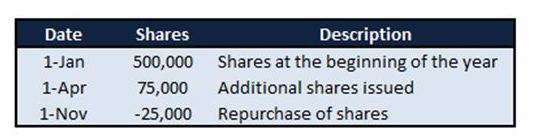# What is Earnings Per Share?

What are the basics of calculating EPS?

One of the more common metrics used when evaluating a company’s profitability is earnings per share.  This ratio, which is mostly used when analyzing equity investments, is simply the portion of a company’s profit that is allocated to each outstanding share of common stock. In this article we will answer the question of what is earnings per share and cover several calculation methods.

Although you should consider other ratios as well, you should definitely look into a company’s EPS before buying their shares. Understanding the different types of earnings per share and when they are used, as well as how earnings per share is calculated, will help investors, as well as those studying for any of the CFA or CPA exams. So, what is earnings per share?

## Weighted Average Shares

No matter what method you are using to calculate EPS, you are going to need to calculate the weighted average number of shares the company has outstanding.  The weighted average number of shares outstanding is simply a time weighted adjustment of the common shares.  It is important to calculate this because instead of just using the number of shares outstanding at the end of a period, time-weighting looks throughout the whole year and takes into consideration any stock splits, reverse stock splits, or share repurchases.  (Stock splits/reverse stock splits are treated as if it has occurred in the beginning of the accounting period.)  Below is an example of how to calculate weighted average number of shares.

Example: A company has 500,000 shares outstanding as of January 1st. At the beginning of April they issue an additional 75,000 shares, and in October they repurchase 25,000 shares.  What is the weighted number of shares the company has outstanding at the end of the year?

To calculate, determine the number of months that are left in the year for each event that will change the number of outstanding shares – record this number as variable n. Next, multiply the share activity by n/12 for each event, and then add all of them up.## Basic EPS

Basic earnings per share is calculated when a company has a simple capital structure, which means that the company has not issued any securities that can increase the company’s number of outstanding shares (the company only issues common stock, and non-convertible preferred stock or debt securities).

To calculate Basic EPS: subtract any preferred dividends from the company’s net income and then divide that by the weighted average number of shares outstanding.

Example: For the year ended 31 December 2014, Peter’s Pizzeria, a nation-wide chain restaurant, had net income of \$750,000.  The company declared and paid \$150,000 of preferred stock dividends, and had the following common stock share events:## What is the company’s basic EPS?

First, calculate the company’s weighted average number of shares outstanding. Using the calculations above, you will determine that the amount is 550,000.  Next, subtract the preferred share dividend of \$150,000 from the \$750,000 net income to arrive at \$600,000.  Lastly, divide the adjusted net income by the number of weighted outstanding shares to arrive at an EPS of \$1.09 ((750,000-150,000)/550,000 = 1.09).

## What is Earnings Per Share – Summary

When calculating EPS, the first step to take is to determine if the company has a simple capital structure – look to see if they have any outstanding financial instruments that could increase the number of outstanding shares such as convertible preferred stock, convertible debt, or any options and warrants. If they do have a simple capital structure, calculate the weighted average number of outstanding shares, subtract any preferred stock dividends from the company’s net income, and then divide that by the weighted average number of outstanding shares.

Look for What is Earnings Per Share: Part 2 to learn about calculating Diluted EPS – the earnings per share of companies with a complex capital structure.

For more investing based articles, check out our investing section.